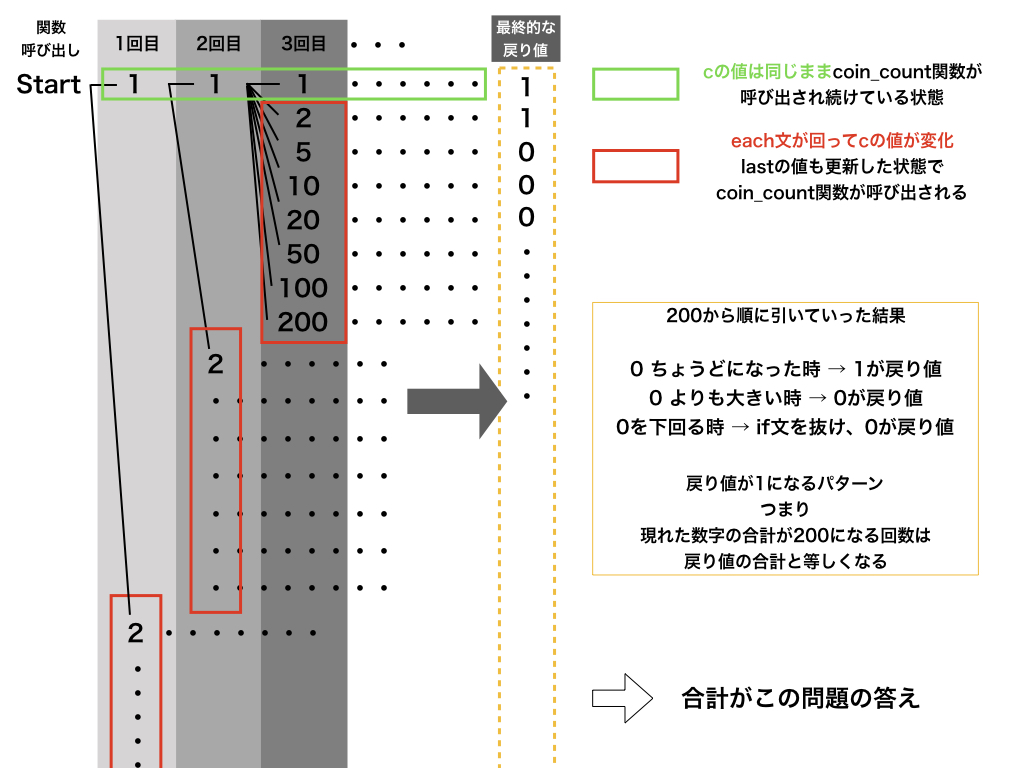# Introduction

About Project Euler's Probem31. A recursive function beginner explained it with reference to other people's answers in the recursive function. Illustration is also shown below, so please take a look there as well.

# problem

Problem 31 "Sum of coins" In the UK, coins are pound sterling and pence p, and there are eight types of coins in circulation: 1p, 2p, 5p, 10p, 20p, 50p, £1 (100p) and £2 (200p). It is possible to make £ 2 by the following method. 1×£1 + 1×50p + 2×20p + 1×5p + 1×2p + 3×1p How many ways are there to make £ 2 with these coins?

# policy

Repeat the process of taking out any one coin from `coins = [1,2,5,10,20,50,100,200]` until it reaches 200. It wouldn't be great if it was done by human hands, but I can't count it, so in such cases, `" recursive function "`!

#### What is a recursive function?

A function that calls itself within the function defined by `def ~ end`. The Fibonacci function is often treated as an introduction to recursive functions if it is famous. If you heard it for the first time, please search.

Another commentary article: [Illustration] Factorial recursive function [Ruby]

``````def coin_count(coins, goal, last = 0)
if goal == 0
return 1
end

total = 0
coins.each do |c|
if c < last
next
end
if goal >= c
total += coin_count(coins, goal - c, c)
end
end

total
end

coins = [1,2,5,10,20,50,100,200]
puts coin_count(coins,200)
``````

I was ashamed to say that I couldn't solve it by myself, so I created it based on here. I will explain this solution in Japanese.

# Commentary

What I want to make this time is the number `200`. So set `goal = 200` and the value of one coin

First, the explanation starts from within the each statement in the function.

``````if c < last
next
end
``````

This is to avoid adding more than the most recent addition, `last`. Since `50 + 50 + 100` and` 50 + 100 + 50` are the same in this problem, only the pattern that adds in ascending order should be adopted. next is the process of skipping the process after next in the block.

Next is the third if statement in a function that uses a recursive function.

``````if goal >= c
total += coin_count(coins, goal - c, c)
end
``````

This time, `c` is one of the elements of`coins = [1,2,5,10,20,50,100,200]`, so` 1 <= c`. So the `goal` passed as an argument to`coin_count (coins, goal, last)`will decrease towards 0 for` goal --c`.

If `c = 50 and goal = 200`, it will be as below.

``````Example)
Goal with recursive function-As c is repeated, the argument goal becomes
First time: 150     ※coin_count(coins, 150, 50)Call with
Second time: 100     ※coin_count(coins, 100, 50)
Third time: 50      ※coin_count(coins, 50, 50)
4th: 0       ※coin_count(coins, 0, 50)
``````

The fourth time the function is called with `coin_count (coins, 0, 50)`

``````if goal == 0
return 1
end
``````

It will be, so finally here,

``````total += coin_count(coins, goal - c, c)
``````

The `coin_count function` of will have a` return value of 1`. At that point, it finally becomes `total + = 1`, and 1 is added.

--When `goal> 0` --When `goal <0`

In this case, `total = 0` remains 0 and is passed to the return value. Finally, the total number of combinations is obtained as the value of total.

# Illustrated

I made a diagram of this state in which the function is called repeatedly.# At the end

Please let me know if there are any missing points, incorrect points, or clearer explanations. Thank you for reading.